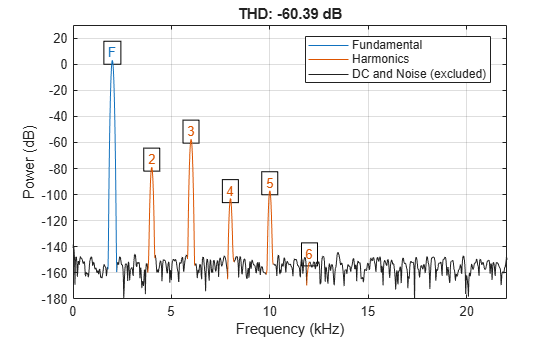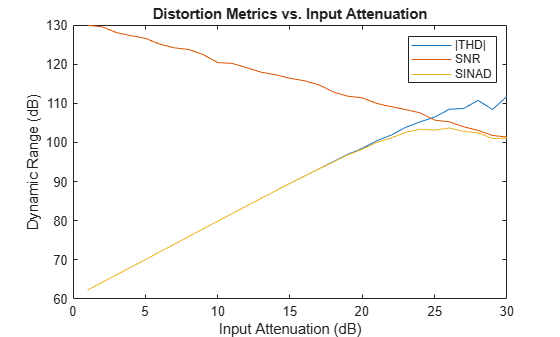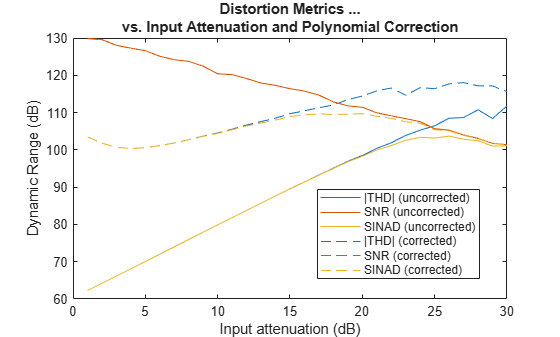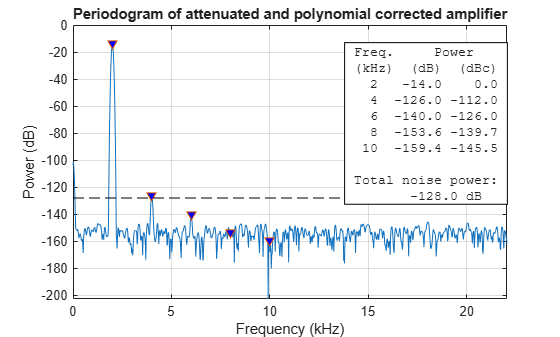Main Content

# Analyzing Harmonic Distortion

This example shows how to analyze the harmonic distortion of a weakly non-linear system in the presence of noise.

### Introduction

In this example we will explore the output of a simplified model of an amplifier that has noise coupled to the input signal and exhibits non-linearity. We will explore how attenuation at the input can reduce harmonic distortion. We will also give an example of how to mathematically correct for the distortion at the output of the amplifier.

### Viewing the Effects of Non-Linearity

A convenient way to view the effect of the non-linearity of the amplifier is to view the periodogram of its output when stimulated with a sinusoid. The amplitude of the sinusoid is set to the maximum allowable voltage of the amplifier. (2 Vpk)

In this example we will source a 2 kHz sinusoid for a duration of 50ms.

```VmaxPk = 2; % Maximum operating voltage Fi = 2000; % Sinusoidal frequency of 2 kHz Fs = 44.1e3; % Sample rate of 44.1kHz Tstop = 50e-3; % Duration of sinusoid t = 0:1/Fs:Tstop; % Input time vector % Use the maximum allowable voltage of the amplifier inputVmax = VmaxPk*sin(2*pi*Fi*t); outputVmax = helperHarmonicDistortionAmplifier(inputVmax);```

View a zoomed-in region of the output sinusoid. Note that the imperfections of our amplifier are difficult to see visually when plotted with respect to time.

```plot(t, outputVmax) xlabel('Time') ylabel('Output Voltage') axis([0 5e-3 -2.5 2.5]) title('Amplifier output')```Now let's view the periodogram of our amplifier output.

`helperPlotPeriodogram(outputVmax, Fs, 'power','annotate');`Note that instead of seeing just the 2 kHz sinusoid that we placed at the input, we see other sinusoids at 4 kHz, 6 kHz, 8 kHz, and 10 kHz. These sinusoids are multiples of the fundamental 2 kHz frequency and are due to the non-linearity of the amplifier.

We also see a relatively flat band of noise power.

### Quantifying Non-Linear Distortion

Let's examine some common distortion metrics for comparison purposes

Our periodogram shows some very well defined harmonics of the fundamental signal. This suggests we measure the total harmonic distortion of the input signal which returns the ratio of power of all harmonic content to the fundamental signal.

`thd(outputVmax, Fs)````ans = -60.3888 ```

Notice that the third and largest harmonic is about 60 dB down from the fundamental. This is where most of the distortion is occurring.

We can also obtain an estimate of the total noise present in our input. To do this, we call SNR which returns the ratio of the power of the fundamental to the power of all non-harmonic content.

`snr(outputVmax, Fs)````ans = 130.9300 ```

Another useful metric to compute is SINAD. This computes the ratio of the power to all other harmonic and noise content in the signal.

`sinad(outputVmax, Fs)````ans = 60.3888 ```

The THD, SNR, and SINAD were -60 dB, 131 dB and 60 dB, respectively. Since the magnitude of THD is roughly equal to SINAD, we can attribute that most of the distortion is due to harmonic distortion.

If we inspect the periodogram, we can notice that the third harmonic dominates the distortion of the output.

### Input Attenuation to Reduce Harmonic Distortion

Most analog circuitry performing amplification has an inherent trade-off between harmonic distortion and noise power. In our example, our amplifier has relatively low noise power compared to the harmonic distortion. This makes it suitable for detecting low power signals. If our input can be attenuated to enter this low-power region, we can recover some of the harmonic distortion.

Let's repeat the measurements by lowering the input voltage by a factor of two.

```inputVhalf = (VmaxPk/2) * sin(2*pi*Fi*t); outputVhalf = helperHarmonicDistortionAmplifier(inputVhalf); helperPlotPeriodogram(outputVhalf, Fs, 'power','annotate');```Let's redo our metrics again, this time measuring the effect of lowering the input voltage.

`thdVhalf = thd(outputVhalf, Fs)`
```thdVhalf = -72.0676 ```
`snrVhalf = snr(outputVhalf, Fs)`
```snrVhalf = 124.8767 ```
`sinadVhalf = sinad(outputVhalf, Fs)`
```sinadVhalf = 72.0676 ```

Notice that simply attenuating the input power level by 6 dB reduces the harmonic content. The SINAD and THD improved from ~60 dB to ~72 dB. This came at the expense of lowering the SNR from 131 dB to 125 dB.

### SNR THD and SINAD as a Function of Input Attenuation

Can further attenuation improve our overall distortion performance? Let's plot THD, SNR and SINAD as a function of input attenuation, sweeping the input attenuator from 1 to 30 dB.

```% Allocate a table with 30 entries nReadings = 30; distortionTable = zeros(nReadings, 3); % Compute the THD, SNR and SINAD for each of the attenuation settings for i = 1:nReadings inputVbestAtten = db2mag(-i) * VmaxPk * sin(2*pi*Fi*t); outputVbestAtten = helperHarmonicDistortionAmplifier(inputVbestAtten); distortionTable(i,:) = [abs(thd(outputVbestAtten, Fs)) snr(outputVbestAtten, Fs) sinad(outputVbestAtten, Fs)]; end % Plot results plot(distortionTable) xlabel('Input Attenuation (dB)') ylabel('Dynamic Range (dB)') legend('|THD|','SNR','SINAD','Location','best') title('Distortion Metrics vs. Input Attenuation')```The graph shows the usable dynamic range corresponding to each metric. The magnitude of THD corresponds to the range that is free of harmonics. Similarly, SNR corresponds to the dynamic range of that is unaffected by noise; SINAD corresponds to the total dynamic range that is free of distortion.

As can be seen from the graph, SNR degrades as input power attenuation increases. This is because when you attenuate the signal, only the signal is attenuated, but the noise floor of the amplifier stays the same.

Also note that the magnitude of the total harmonic distortion improves steadily until it intersects the SNR curve, after which the measurement becomes unstable. This happens when the harmonics have "disappeared" beneath the noise of the amplifier.

A practical choice of amplifier attenuation for the amplifier would be 26 dB (yielding a SINAD of 103 dB). This would be a reasonable tradeoff between harmonic and noise distortion.

```% Search the table for the largest SINAD reading [maxSINAD, iAtten] = max(distortionTable(:,3)); fprintf('Max SINAD (%.1f dB) occurs at %.f dB attenuation\n', ... maxSINAD, iAtten)```
```Max SINAD (103.7 dB) occurs at 26 dB attenuation ```

Let's plot the periodogram when the attenuator is set to 26 dB.

```inputVbestAtten = db2mag(-iAtten) * VmaxPk * sin(2*pi*Fi*t); outputVbestAtten = helperHarmonicDistortionAmplifier(inputVbestAtten); helperPlotPeriodogram(outputVbestAtten, Fs, 'power','annotate','shownoise');```Here we have additionally plotted the level of total noise power that is spread across the spectrum. Note that at this attenuation setting, the second and third harmonic are still visible in the spectrum but also considerably less than the total noise power. If we were to have an application that uses a smaller bandwidth of the available spectrum we would benefit from further increasing the attenuation to reduce the harmonic content.

### Post-processing to Remove Distortion

Occasionally we can correct for some of the non-linearity of the amplifier. If the output of the amplifier is digitized, we can recover more useful dynamic range by digitally post-processing the captured output and correcting for the non-linearity mathematically.

In our case, we stimulate the input with a linear ramp and fit a third-order polynomial that best fits the input.

```inputRamp = -2:0.00001:2; outputRamp = helperHarmonicDistortionAmplifier(inputRamp); polyCoeff = polyfit(outputRamp,inputRamp,3)```
```polyCoeff = 1×4 0.0010 -0.0002 1.0000 -0.0250 ```

Now that we have the coefficients we can then perform post-correction at the output and compare side-by-side with our original uncorrected output

```correctedOutputVmax = polyval(polyCoeff, outputVmax); helperPlotPeriodogram([outputVmax; correctedOutputVmax],Fs,'power'); subplot(2,1,1) title('Uncorrected') subplot(2,1,2) title('Polynomial Corrected')```Note that the second and third harmonics are significantly reduced when using polynomial correction.

Let's repeat the measurements again with the corrected output.

`thdCorrectedVmax = thd(correctedOutputVmax, Fs)`
```thdCorrectedVmax = -99.6194 ```
`snrCorrectedVmax = snr(correctedOutputVmax, Fs)`
```snrCorrectedVmax = 130.7491 ```
`sinadCorrectedVmax = sinad(correctedOutputVmax, Fs)`
```sinadCorrectedVmax = 99.6162 ```

Notice that our SINAD (and THD) dropped from 60 dB down to 99 dB, while preserving our original SNR of 131 dB.

### Combining Techniques

We can combine attenuation with polynomial evaluation to find the ideal operating voltage that minimizes the overall SINAD of our system.

```subplot(1,1,1) % Add three more columns to our distortion table distortionTable = [distortionTable zeros(nReadings,3)]; for i = 1:nReadings inputVreduced = db2mag(-i) * VmaxPk * sin(2*pi*Fi*t); outputVreduced = helperHarmonicDistortionAmplifier(inputVreduced); correctedOutput = polyval(polyCoeff, outputVreduced); distortionTable(i,4:6) = [abs(thd(correctedOutput, Fs)) snr(correctedOutput, Fs) sinad(correctedOutput, Fs)]; end h = plot(distortionTable)```
```h = 6x1 Line array: Line Line Line Line Line Line ```
```xlabel('Input attenuation (dB)') ylabel('Dynamic Range (dB)') for i = 1:3 h(i+3).Color = h(i).Color; h(i+3).LineStyle = '--' ; end legend('|THD| (uncorrected)','SNR (uncorrected)','SINAD (uncorrected)', ... '|THD| (corrected)','SNR (corrected)','SINAD (corrected)','Location','best') title('Distortion Metrics vs. Input Attenuation and Polynomial Correction');```Here, we've plotted all three metrics alongside for both the uncorrected and polynomial corrected amplifier.

As can be seen from the graph, THD has improved considerably, whereas SNR was not affected by polynomial correction. This is to be expected since the polynomial correction only affects the harmonic distortion and not the noise distortion.

Let's show the maximum SINAD possible when corrected by the polynomial

```[maxSINADcorrected, iAttenCorr] = max(distortionTable(:,6)); fprintf('Corrected: Max SINAD (%.1f dB) at %.f dB attenuation\n', ... maxSINADcorrected, iAttenCorr)```
```Corrected: Max SINAD (109.7 dB) at 17 dB attenuation ```

A good choice of amplifier attenuation for the polynomial corrected amplifier would be 20dB (yielding a SINAD of 109.8 dB).

```% Recompute amplifier at maximum SINAD attenuation setting with polynomial inputVreduced = db2mag(-iAttenCorr) * VmaxPk * sin(2*pi*Fi*t); outputVreduced = helperHarmonicDistortionAmplifier(inputVreduced); correctedOutputVbestAtten = polyval(polyCoeff, outputVreduced); helperPlotPeriodogram(correctedOutputVbestAtten, Fs, 'power','annotate','shownoise'); title('Periodogram of attenuated and polynomial corrected amplifier')```Note that all but the second harmonic disappeared entirely with polynomial correction under the ideal attenuation setting. As noted before the second harmonic appears just underneath the power level of the total noisefloor. This provides a reasonable tradeoff in applications which use the full bandwidth of the amplifier.

### Summary

We have shown how polynomial correction can be applied to the output of an amplifier experiencing distortion and how to pick a reasonable attenuation value to reduce the effects of harmonic distortion.

## Support

#### Deep Learning for Signal Processing with MATLAB

Download white paper